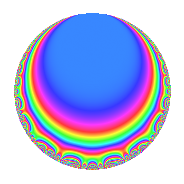# Properties

 Label 4005.2.a.iLevel 4005 Weight 2 Character orbit 4005.a Self dual Yes Analytic conductor 31.980 Analytic rank 0 Dimension 3 CM No Inner twists 1

# Related objects

## Newspace parameters

 Level: $$N$$ = $$4005 = 3^{2} \cdot 5 \cdot 89$$ Weight: $$k$$ = $$2$$ Character orbit: $$[\chi]$$ = 4005.a (trivial)

## Newform invariants

 Self dual: Yes Analytic conductor: $$31.9800860095$$ Analytic rank: $$0$$ Dimension: $$3$$ Coefficient field: $$\Q(\zeta_{18})^+$$ Coefficient ring: $$\Z[a_1, a_2]$$ Coefficient ring index: $$1$$ Fricke sign: $$-1$$ Sato-Tate group: $\mathrm{SU}(2)$

## $q$-expansion

Coefficients of the $$q$$-expansion are expressed in terms of a basis $$1,\beta_1,\beta_2$$ for the coefficient ring described below. We also show the integral $$q$$-expansion of the trace form.

 $$f(q)$$ $$=$$ $$q + \beta_{1} q^{2} + \beta_{2} q^{4} - q^{5} + ( 1 + \beta_{2} ) q^{7} + ( 1 - \beta_{1} ) q^{8} +O(q^{10})$$ $$q + \beta_{1} q^{2} + \beta_{2} q^{4} - q^{5} + ( 1 + \beta_{2} ) q^{7} + ( 1 - \beta_{1} ) q^{8} -\beta_{1} q^{10} + ( 2 - 2 \beta_{1} + 2 \beta_{2} ) q^{11} + ( -1 + \beta_{1} - \beta_{2} ) q^{13} + ( 1 + 2 \beta_{1} ) q^{14} + ( -2 + \beta_{1} - 3 \beta_{2} ) q^{16} + ( 3 + \beta_{1} ) q^{17} -2 \beta_{1} q^{19} -\beta_{2} q^{20} + ( -2 + 4 \beta_{1} - 2 \beta_{2} ) q^{22} + ( -2 \beta_{1} + 4 \beta_{2} ) q^{23} + q^{25} + ( 1 - 2 \beta_{1} + \beta_{2} ) q^{26} + ( 2 + \beta_{1} ) q^{28} + ( 5 + \beta_{1} - 2 \beta_{2} ) q^{29} + ( -2 + 4 \beta_{2} ) q^{31} + ( -3 - 3 \beta_{1} + \beta_{2} ) q^{32} + ( 2 + 3 \beta_{1} + \beta_{2} ) q^{34} + ( -1 - \beta_{2} ) q^{35} + ( -1 + \beta_{1} + 4 \beta_{2} ) q^{37} + ( -4 - 2 \beta_{2} ) q^{38} + ( -1 + \beta_{1} ) q^{40} + ( 7 - 2 \beta_{1} + 3 \beta_{2} ) q^{41} + ( 3 - 3 \beta_{1} + 2 \beta_{2} ) q^{43} + 2 q^{44} + ( 4 \beta_{1} - 2 \beta_{2} ) q^{46} + ( 3 - \beta_{2} ) q^{47} + ( -4 + \beta_{1} + \beta_{2} ) q^{49} + \beta_{1} q^{50} - q^{52} + ( -3 - 2 \beta_{1} + 3 \beta_{2} ) q^{53} + ( -2 + 2 \beta_{1} - 2 \beta_{2} ) q^{55} + ( -2 \beta_{1} + \beta_{2} ) q^{56} + ( 3 \beta_{1} + \beta_{2} ) q^{58} + ( 3 + \beta_{1} + 3 \beta_{2} ) q^{59} -2 \beta_{1} q^{61} + ( 4 + 2 \beta_{1} ) q^{62} + ( -1 - 4 \beta_{1} + 3 \beta_{2} ) q^{64} + ( 1 - \beta_{1} + \beta_{2} ) q^{65} + ( -2 + 4 \beta_{1} + 2 \beta_{2} ) q^{67} + ( 1 + \beta_{1} + 3 \beta_{2} ) q^{68} + ( -1 - 2 \beta_{1} ) q^{70} + ( 4 + 8 \beta_{1} - 8 \beta_{2} ) q^{71} + ( -2 + 2 \beta_{1} - 4 \beta_{2} ) q^{73} + ( 6 + 3 \beta_{1} + \beta_{2} ) q^{74} + ( -2 - 2 \beta_{1} ) q^{76} + ( 4 - 2 \beta_{1} + 2 \beta_{2} ) q^{77} + ( -1 - 4 \beta_{1} + \beta_{2} ) q^{79} + ( 2 - \beta_{1} + 3 \beta_{2} ) q^{80} + ( -1 + 10 \beta_{1} - 2 \beta_{2} ) q^{82} + ( 4 + 2 \beta_{1} - 6 \beta_{2} ) q^{83} + ( -3 - \beta_{1} ) q^{85} + ( -4 + 5 \beta_{1} - 3 \beta_{2} ) q^{86} + ( 4 - 6 \beta_{1} + 4 \beta_{2} ) q^{88} - q^{89} + ( -2 + \beta_{1} - \beta_{2} ) q^{91} + ( 6 + 2 \beta_{1} - 4 \beta_{2} ) q^{92} + ( -1 + 2 \beta_{1} ) q^{94} + 2 \beta_{1} q^{95} + ( 4 \beta_{1} + 2 \beta_{2} ) q^{97} + ( 3 - 3 \beta_{1} + \beta_{2} ) q^{98} +O(q^{100})$$ $$\operatorname{Tr}(f)(q)$$ $$=$$ $$3q - 3q^{5} + 3q^{7} + 3q^{8} + O(q^{10})$$ $$3q - 3q^{5} + 3q^{7} + 3q^{8} + 6q^{11} - 3q^{13} + 3q^{14} - 6q^{16} + 9q^{17} - 6q^{22} + 3q^{25} + 3q^{26} + 6q^{28} + 15q^{29} - 6q^{31} - 9q^{32} + 6q^{34} - 3q^{35} - 3q^{37} - 12q^{38} - 3q^{40} + 21q^{41} + 9q^{43} + 6q^{44} + 9q^{47} - 12q^{49} - 3q^{52} - 9q^{53} - 6q^{55} + 9q^{59} + 12q^{62} - 3q^{64} + 3q^{65} - 6q^{67} + 3q^{68} - 3q^{70} + 12q^{71} - 6q^{73} + 18q^{74} - 6q^{76} + 12q^{77} - 3q^{79} + 6q^{80} - 3q^{82} + 12q^{83} - 9q^{85} - 12q^{86} + 12q^{88} - 3q^{89} - 6q^{91} + 18q^{92} - 3q^{94} + 9q^{98} + O(q^{100})$$

Basis of coefficient ring in terms of $$\nu = \zeta_{18} + \zeta_{18}^{-1}$$:

 $$\beta_{0}$$ $$=$$ $$1$$ $$\beta_{1}$$ $$=$$ $$\nu$$ $$\beta_{2}$$ $$=$$ $$\nu^{2} - 2$$
 $$1$$ $$=$$ $$\beta_0$$ $$\nu$$ $$=$$ $$\beta_{1}$$ $$\nu^{2}$$ $$=$$ $$\beta_{2} + 2$$

## Embeddings

For each embedding $$\iota_m$$ of the coefficient field, the values $$\iota_m(a_n)$$ are shown below.

For more information on an embedded modular form you can click on its label.

Label $$\iota_m(\nu)$$ $$a_{2}$$ $$a_{3}$$ $$a_{4}$$ $$a_{5}$$ $$a_{6}$$ $$a_{7}$$ $$a_{8}$$ $$a_{9}$$ $$a_{10}$$
1.1
 −1.53209 −0.347296 1.87939
−1.53209 0 0.347296 −1.00000 0 1.34730 2.53209 0 1.53209
1.2 −0.347296 0 −1.87939 −1.00000 0 −0.879385 1.34730 0 0.347296
1.3 1.87939 0 1.53209 −1.00000 0 2.53209 −0.879385 0 −1.87939
 $$n$$: e.g. 2-40 or 990-1000 Significant digits: Format: Complex embeddings Normalized embeddings Satake parameters Satake angles

## Inner twists

This newform does not admit any (nontrivial) inner twists.

## Atkin-Lehner signs

$$p$$ Sign
$$3$$ $$-1$$
$$5$$ $$1$$
$$89$$ $$1$$

## Hecke kernels

This newform can be constructed as the intersection of the kernels of the following linear operators acting on $$S_{2}^{\mathrm{new}}(\Gamma_0(4005))$$:

 $$T_{2}^{3} - 3 T_{2} - 1$$ $$T_{7}^{3} - 3 T_{7}^{2} + 3$$ $$T_{11}^{3} - 6 T_{11}^{2} + 8$$